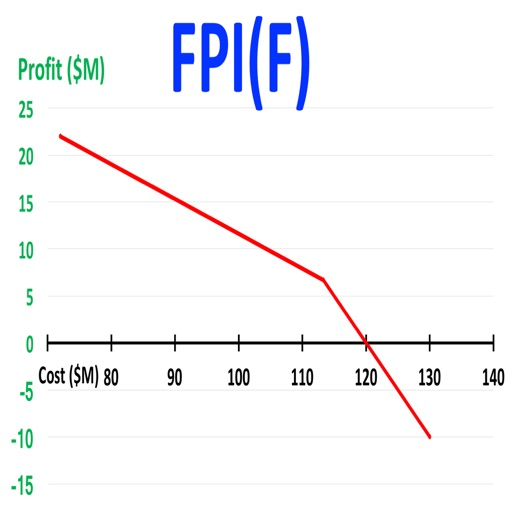## FPIF Calculator takes standard inputs for a fixed price incentive (firm target) government contract (target cost, target profit percentage, ceiling price percentage, and government/contractor share ratio) and calculates the target price, ceiling price, point of total assumption (which is cost where the FPI(F) contract converts to a fixed price contract), and the profit at the point of total assumption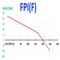# FPIF Calculator

by Neil Whiteman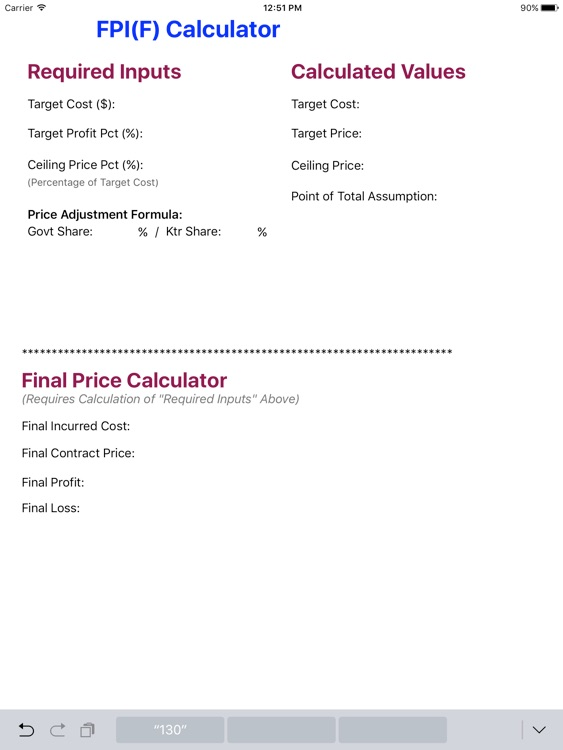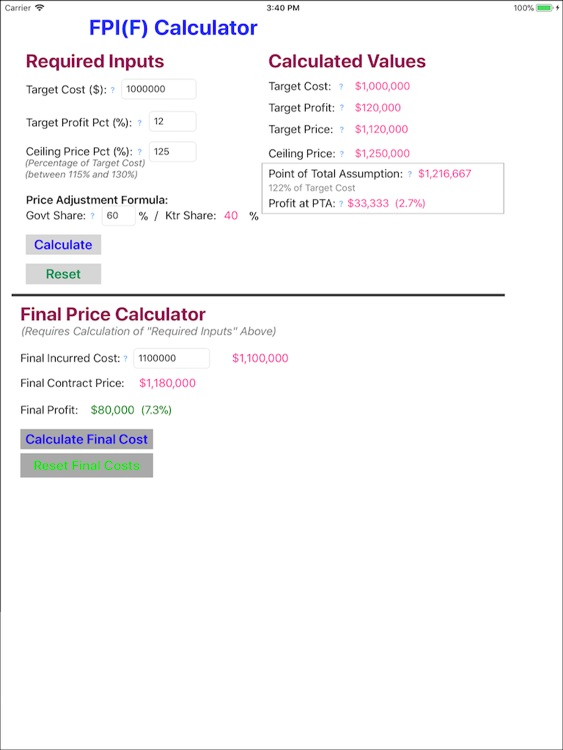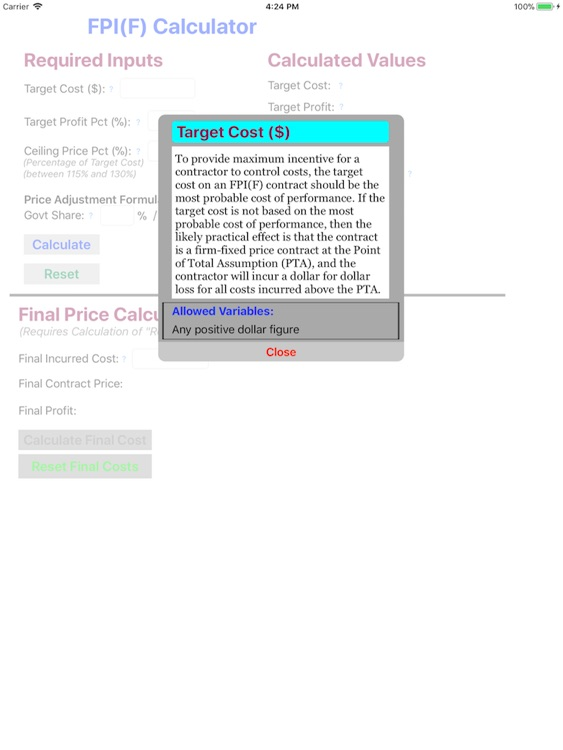FPIF Calculator takes standard inputs for a fixed price incentive (firm target) government contract (target cost, target profit percentage, ceiling price percentage, and government/contractor share ratio) and calculates the target price, ceiling price, point of total assumption (which is cost where the FPI(F) contract converts to a fixed price contract), and the profit at the point of total assumption. FPIF Calculator also calculates the final cost of an FPI(F) contract based on the incurred costs. This app easily performs your complex FPI(F) contract calculations and enables quick comparison of various contract geometry scenarios. The ability to rapidly calculate and compare various FPI(F) contract geometry scenarios facilitates enhanced understanding of the tradeoff alternatives and inherent values embedded in every FPI(F) contract. Reference: FAR 52.216-16, Incentive Price Revision—Firm Target (Oct. 1997).Version
1.25
Rating
NA
Size
14Mb
Genre
Last updated
August 23, 2018
Release date
August 7, 2017

### App Screenshots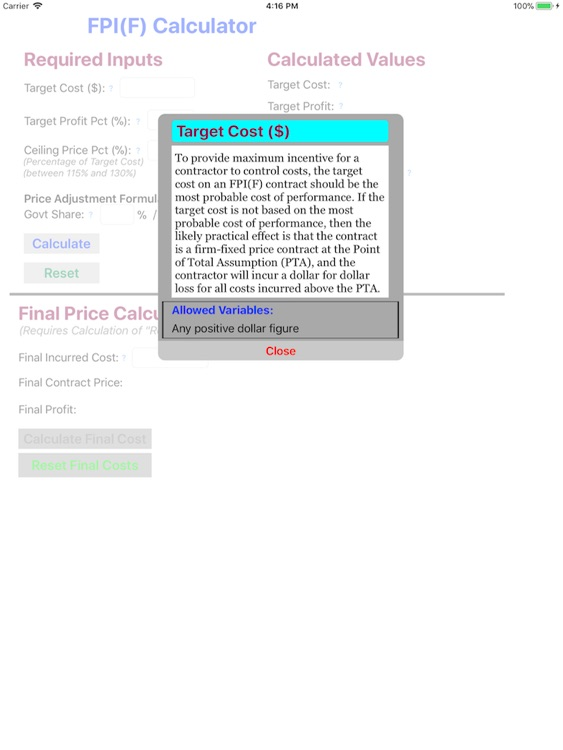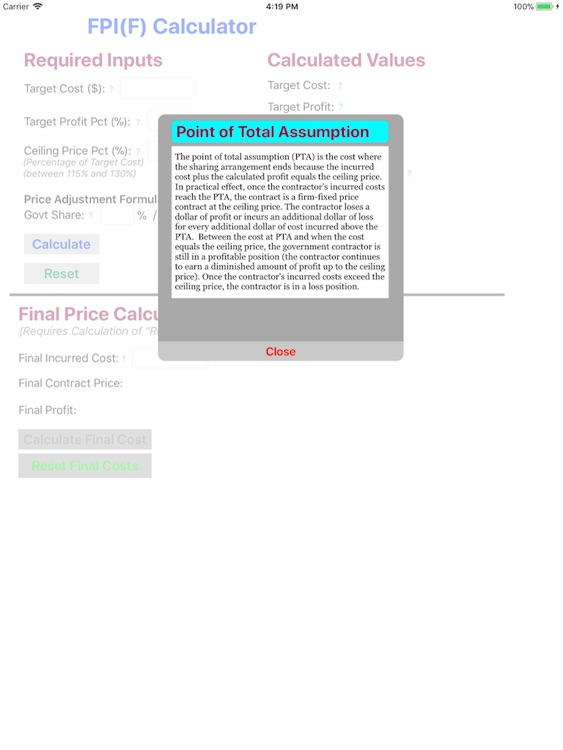### App Store Description

FPIF Calculator takes standard inputs for a fixed price incentive (firm target) government contract (target cost, target profit percentage, ceiling price percentage, and government/contractor share ratio) and calculates the target price, ceiling price, point of total assumption (which is cost where the FPI(F) contract converts to a fixed price contract), and the profit at the point of total assumption. FPIF Calculator also calculates the final cost of an FPI(F) contract based on the incurred costs. This app easily performs your complex FPI(F) contract calculations and enables quick comparison of various contract geometry scenarios. The ability to rapidly calculate and compare various FPI(F) contract geometry scenarios facilitates enhanced understanding of the tradeoff alternatives and inherent values embedded in every FPI(F) contract. Reference: FAR 52.216-16, Incentive Price Revision—Firm Target (Oct. 1997).

Keep your eye out for future versions of this app that will graph the FPI(F) contract geometry.

Disclaimer:
AppAdvice does not own this application and only provides images and links contained in the iTunes Search API, to help our users find the best apps to download. If you are the developer of this app and would like your information removed, please send a request to [email protected] and your information will be removed.